+

# Formulas

Author: Sophia Tutorial
##### Description:

Identify the correct formula for a given shape.

(more)

Sophia’s self-paced online courses are a great way to save time and money as you earn credits eligible for transfer to many different colleges and universities.*

No credit card required

37 Sophia partners guarantee credit transfer.

299 Institutions have accepted or given pre-approval for credit transfer.

* The American Council on Education's College Credit Recommendation Service (ACE Credit®) has evaluated and recommended college credit for 33 of Sophia’s online courses. Many different colleges and universities consider ACE CREDIT recommendations in determining the applicability to their course and degree programs.

Tutorial
what's covered
1. Definition of Formulas
2. Examples of Common Formulas
3. Substitution in Formulas

# 1. Definition of Formulas

Formulas are often times thought of as special types of equations where two or more quantities are equated to one another.

term to know
Formula
A mathematical rule that relates two or more quantities.

There are several different types of formulas that we will come across when working in most math courses. Here are a few of the most common formulas.

# 2. Examples of Common Formulas

formula
Area of a Rectangle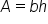, where A is the area, b is the base, and h is the height.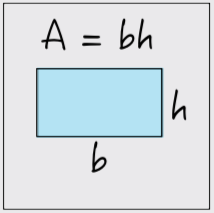formula
Area of a Triangle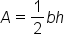, where A is area, b is base, and h is height.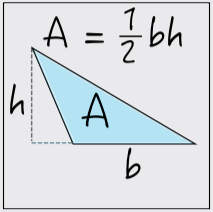formula
Area of a Circle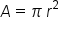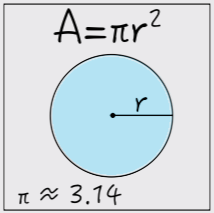formula
Volume of a Rectangular Prism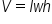, where V is volume, l is length, w is width, and h is height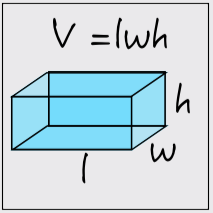formula
Volume of a Cylinder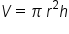, where V is volume, r is the radius of the circular base, h is height, and π is approximately 3.14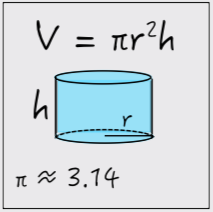formula
Volume of a Sphere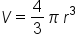, where V is volume, r is the radius, and π is approximately 3.14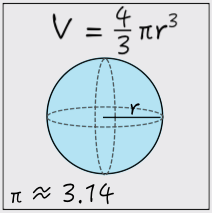formula
Pythagorean Theorem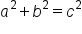, where a and b are the vertical and horizontal legs of the triangle, and c is the hypotenuse of the right triangle (opposite the right angle).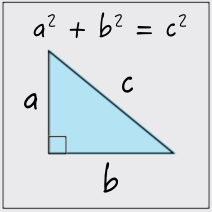formula
Compound Interest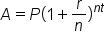, where A is the account balance, P is the principal (initial) balance, r is the interest rate (expressed as a decimal), n is the number of times per year the interest is compounded, and t is time in years.

# 3. Substitution in Formulas

Formulas become most handy when we are given the value of several quantities and asked to determine the value of another quantity. For example, suppose we are told that a rectangle has an area of 40 square feet and a base of 4 feet. What would the height of the rectangle be?

To calculate the length of the rectangle, we can take the area, A, and base, b, and substitute them in the formula for a rectangle’s area. We can then solve for the height, h.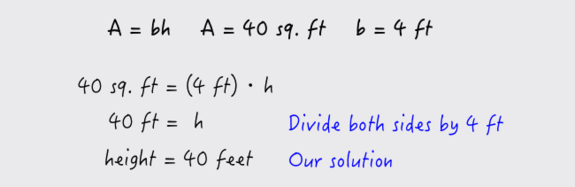When we wish to tell whether or not we can use a formula in this way, we simply need to check that we have a value of all but one variable in the formula. If that is the case then we can solve for the unknown quantity using the appropriate formula.

summary
The definition of formulas states that formulas are used to relate two or more different quantities. There are many examples of common formulas, such as area of a rectangle or triangle, or the volume of a sphere or cylinder. We most often use formulas when we are given one or more of those variables, and we need to solve for an unknown variable, or something that we want to find. This is done by substituting values for known variables into our formula and then evaluating the formula to find the unknown variable.

Terms to Know
Equation

A mathematical statement that two expressions or quantities have the same value.

Formula

A mathematical rule that relates two or more quantities.

Variable

A symbol (usually a letter) used to represent a value that can change.

Formulas to Know
Area of CircleArea of RectangleArea of TriangleCompound InterestVolume of CylinderVolume of Rectangular PrismVolume of SphereRating